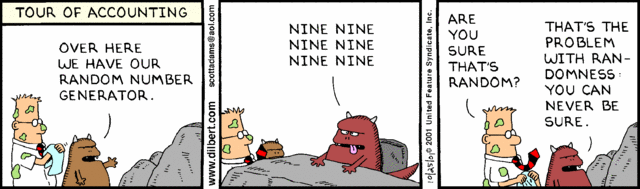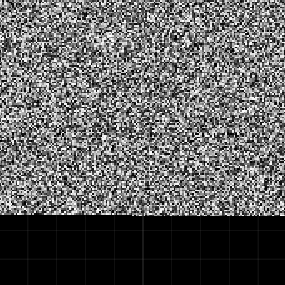# How to generate random numbers in Unity with C# and shader code

## 1. Generate random numbers in Unity with C#

Generating pseudo random numbers in Unity is easy. This is how it works:

```Random.InitState(12345); //This is the seed

print(Random.Range(0, 10)); //returns 6 every time
print(Random.Range(0, 10)); //returns 6 every time
print(Random.Range(0, 10)); //returns 9 every time
```

One of properties of a true random number is that you shouldn't be able to find a pattern between the numbers. Another property of a true random number is that nobody should be able to reconstruct the sequence at a later time. But with pseudo random numbers you will be able to generate the same sequence of random numbers because you are using a so-called seed. You always need a seed to start the algorithm. If you are just writing Random.Range(0, 10), Unity is using some built-in seed to generate the number. This seed can come from different sources:

• From the system clock when the program is launched or when the game begins.
• From the frequency of the user's keystrokes or mouse movement when the program is launched or when the game begins.

If you are using a known seed you can replicate whatever you have done. I recall a Kaggle competition where you are competing in who can build the best model to predict something from data. Someone came up with the winning model and this model used pseudo random numbers. But he/she couldn't replicate the winning model because when the person tried to again run the model, the new random numbers resulted in a worse model. If that person had used a known seed, the model would have generated the same random numbers as in the winning model. In the end someone else won the money. So always use a known seed!

What the pseudo random number generators have in common is that they are a special type of function known as a recurrence relation. This means that a the value at a given time step n is dependent on values from previous steps. The simplest example is the Fibonacci series:

```//Generate random numbers with the Fibonacci series
//The seed should be 0, 1
void GetRandomFibonacci(int xMinusOneSeed, int xMinusTwoSeed)
{
int xMinusOne = xMinusOneSeed;

int xMinusTwo = xMinusTwoSeed;

//The Fibonacci series is endless so we have to limit by using a modulus
int m = int.MaxValue;

string allRandomNumbers = "";

for (int i = 0; i < 100; i++)
{
//x_n = x_(n-1) + x_(n-2)

//Numbers in C# wrap, so if the sum is larger than the possible value, it begins
//from the smallest possible value, so we have to check if the sum is < 0
int sum = xMinusOne + xMinusTwo;

if (xMinusOne + xMinusTwo < 0)
{
sum = int.MaxValue;
}

int randomNumber = sum % m;

allRandomNumbers += randomNumber.ToString() + " ";

xMinusTwo = xMinusOne;
xMinusOne = randomNumber;
}

print(allRandomNumbers);
}
```

### How can we test that numbers generated by a PRNG are random?

The Fibonacci series is a useless random number generator because the sequence is 0, 1, 1, 2, 3, 5, 8, 13, 21, ... So someone who knows the Fibonacci series can figure out the next number. Moreover, the sequence is stabilizing and repeats the sequence 0, 1836311903, 1836311903, 0, 1836311903, ... over and over again. This is called the random generator's period, which is how long it takes before the numbers starts repeating. So we will need something better because the period is way too low, but the Fibonacci series is a good example of the basic ideas behind how to generate random numbers.How can we test that the pseudo random number generator is producing random numbers? The easiest way is to visualize the random numbers on the screen in the form of a grid where each square is a gray-scale color. So we need a way to convert from whatever random value we have to a range between 0 and 1. This method is doing exactly that:

```//Convert to the correct range
//http://stackoverflow.com/questions/929103/convert-a-number-range-to-another-range-maintaining-ratio
//originalNumber is the number we want to convert
//oldMin and oldMax is the range of the random numbers we have
//newMin and newMax is the range we want, such as 0, 1
float ConvertToRange(float originalNumber, float oldMin, float oldMax, float newMin, float newMax)
{
float oldRange = oldMax - oldMin;
float newRange = newMax - newMin;

float convertedNumber = (((originalNumber - oldMin) * newRange) / oldRange) + newMin;

return convertedNumber;
}
```

We humans are really good at spotting patterns, and visualisation allows us to use our eyes and brain to try to see if the pattern is random. This is not the best method, but it's the easiest and fastest method. A more complicated but better way is the Diehard test, which is a battery of statistical tests for measuring the quality of a random number generator.

To be able to visualize the random numbers on the screen you need to create a plane, give the plane a material with an Unlit/Texture shader and drag it to the script after you've added the following to the top of the script:

```//A plane to which we will add a texture showing how random a random generator is
public Renderer textureRenderer;
```

This method will generate the texture and add it to the material that's added to the plane you just added:

```//Display the noise on the screen for debugging
void DrawRandomMap(float[] randomValues)
{
//The random values are 1D, but we want to display them in a square
int width = Mathf.FloorToInt(Mathf.Sqrt(randomValues.Length));

//From random values to colors
Color[] colorMap = new Color[width * width];

for (int x = 0; x < width * width; x++)
{
//The colors are gray scale
colorMap[x] = Color.Lerp(Color.black, Color.white, randomValues[x]);
}

//Add the colors to the texture
Texture2D texture = new Texture2D(width, width);

texture.SetPixels(colorMap);

//Remove the blur from the texture
texture.filterMode = FilterMode.Point;

texture.wrapMode = TextureWrapMode.Clamp;

texture.Apply();

//Add the texture to the material
textureRenderer.material.mainTexture = texture;
}
```

### What are some pseudo random numbers algorithms?

What exactly is happening when we write Random.Range(0, 10) and get back a "random" integer in the range 0 to 10? The answer is that there's some kind of algorithm that's generating a number. I'm not sure what algorithm is used to generate Unity's random numbers, but C#'s Random class is, according to the documentation, using an algorithm based on a modified version of Donald E. Knuth's subtractive random number generator algorithm. So there's more than one type of algorithm generating pseudo random numbers. If you study the area you will learn that the Mersenne Twister is considered the best pseudo random number generator.

The Middle Square Method. Was suggested by no-one else than the famous mathematician John von Neumann himself. But even the sun has spots because the method is not a good method. The period is usually very short and it has some severe weaknesses, such as the output sequence almost always converging to zero. But it's easy to implement. The basic idea is that you multiply the seed with itself, add 0s to the seed if it is shorter than the double of the amount of digits in the seed. Then you take the middle of this seed, and this is your random number. To generate another random number, you just use the random number you got as a seed.

```//Generate random numbers with the Middle Square Method
void GetRandomMiddleSquare()
{
//We cant use the regular int32, which max value is 2147483647
//So we have to use long, which max value is 9,223,372,036,854,775,807
long seed = 5234567890;

//How many digits in the seed?
int digits = seed.ToString().Length;

//To display the random numbers when the loop is finished
string allRandomNumbers = "";

//How many random numbers do we want to generate?
int amountOfNumbers = 1000;

//Array that will store the random numbers so we can display them
float[] randomNumbers = new float[amountOfNumbers];

for (int i = 0; i < amountOfNumbers; i++)
{
//Square the seed
long seedSqr = seed * seed;

//Make it a string
string randomString = seedSqr.ToString();

//If the string has less than digits * 2 characters, add zeros to the front of the string
while (randomString.Length < digits * 2)
{
randomString = 0 + randomString;
}

//Get the middle characters of this string
int start = Mathf.FloorToInt(digits / 2);

string middleCharacters = randomString.Substring(start, digits);

//The next seed in the next loop is these middle characters
seed = long.Parse(middleCharacters);

//If we want a float between 0 and 1 we divide the maximum number with 9999 if we have 4 digits
float divisor = (Mathf.Pow(10f, digits)) - 1f;

//Remove this line if you want to speed up the testing of the algorithm
allRandomNumbers += (seed / divisor) + " ";

randomNumbers[i] = seed / divisor;
}

print(allRandomNumbers);

DrawRandomMap(randomNumbers);
}
```

This is how it looks like if we visualize the Middle Square Method. The result is actually good if you have a 10 digit seed. If the seed is like 5 digits, the algorithm stops generating new random numbers after just a few iterations.But if you are looping the algorithm 30000 times, you will se that it stops working:The Linear Congruential Generator (LCG). Is one of the oldest pseudo random number generators and is the most commonly taught and commonly used RNG, so it's far more common than the Middle Square Method. It's usually the basis for RNGs in most math libraries. If I've understood everything correctly, Donald E. Knuth's subtractive random number generator algorithm, which is the algorithm probably used by Unity, is a Linear Congruential Generator. But the algorithm is far from perfect, so don't use it in cryptographic applications.

In addition to the seed, the algorithm uses three values: m, a, and c. You can find examples of what those values have been in different implemenations of the algorithm on Wikipedia.

```//Generate random numbers with the Linear Congruential Generator
void GetRandomLinearCongruential()
{
long seed = 100;

//Needs the following parameters, which can be found on Wikipedia for different implementations
//https://en.wikipedia.org/wiki/Linear_congruential_generator
//multiplier
long a = 1103515245;
//increment
long c = 12345;
//modulus m (which is also the maximum possible random value)
long m = (long)Mathf.Pow(2f, 31f);

//To display the random numbers when the loop is finished
string allRandomNumbers = "";

//How many random numbers do we want to generate?
int amountOfNumbers = 30000;

//Array that will store the random numbers so we can display them
float[] randomNumbers = new float[amountOfNumbers];

for (int i = 0; i < amountOfNumbers; i++)
{
//Basic idea: seed = (a * seed + c) mod m
seed = (a * seed + c) % m;

//To get a value between 0 and 1
float randomValue = seed / (float)m;

//Remove this line if you want to speed up the testing of the algorithm
allRandomNumbers += randomValue + " ";

randomNumbers[i] = randomValue;
}

print(allRandomNumbers);

DrawRandomMap(randomNumbers);
}
```

And this is the result after 30000 iterations:The Linear Congruential Generator will begin to repeat itself with some parameters, but the repetition is not as obvious as in the Middle Square Method where we got all black.